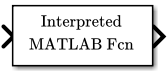# Interpreted MATLAB Function

(To be removed) Apply MATLAB function or expression to input

The Interpreted MATLAB Function block will be removed in a future release. Use built-in blocks instead. For more information on updating your code, see Version History.

• Library:

•## Description

The Interpreted MATLAB Function block applies the specified MATLAB® function or expression to the input. The output of the function must match the output dimensions of the block.

Some valid expressions for this block are:

```sin atan2(u(1), u(2)) u(1)^u(2) ```

Note

This block is slow because it calls the MATLAB parser during each integration step. Consider using built-in blocks such as the Math Function block instead. Alternatively, you can write the function as a MEX-file S-function, then access it using the S-Function block.

## Ports

### Input

expand all

The Interpreted MATLAB Function block accepts one real or complex input of type `double` and generates real or complex output of type `double`, depending on the setting of the Output signal type parameter.

Data Types: `double`

### Output

expand all

The Interpreted MATLAB Function block accepts one real or complex input of type `double` and generates real or complex output of type `double`, depending on the setting of the Output signal type parameter.

Data Types: `double`

## Parameters

expand all

Specify the function or expression. If you specify a function only, it is not necessary to include the input argument in parentheses.

Specify the dimensions of the block output signal, for example, `2` for a two-element vector. The output dimensions must match the dimensions of the value returned by the function or expression in the MATLAB function field.

Specify `-1` to inherit the dimensions from the output of the specified function or expression. To determine the output dimensions, Simulink runs the function or expression once before simulation starts.

Note

If you specify `-1` for this parameter and your function has persistent variables, then the variables might update before the simulation starts. If you need to use persistent variables, consider setting this parameter to a value other than `-1`.

Specify the output signal type of the block as `real`, `complex`, or `auto`. A value of `auto` sets the output type to be the same as the type of the input signal.

Select this check box to output a 2-D array as a 1-D array containing the 2-D array's elements in column-major order.

Note

This parameter is not visible in the block dialog box unless it is explicitly set to a value other than `-1`. To learn more, see Blocks for Which Sample Time Is Not Recommended.

## Block Characteristics

 Data Types `double` Direct Feedthrough `yes` Multidimensional Signals `no` Variable-Size Signals `no` Zero-Crossing Detection `no`

## Version History

Introduced in R2011a

expand all